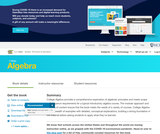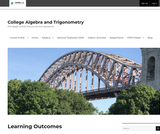# 8 Results

View
Selected filters:
• TrigonometryUnrestricted Use
CC BY
Rating

Algebra and Trigonometry provides a comprehensive exploration of algebraic principles and meets scope and sequence requirements for a typical introductory algebra and trigonometry course. The modular approach and the richness of content ensures that the book meets the needs of a variety of courses. Algebra and Trigonometry offers a wealth of examples with detailed, conceptual explanations, building a strong foundation in the material before asking students to apply what they’ve learned.

Subject:
Algebra
Trigonometry
Material Type:
Textbook
Provider:
Rice University
Provider Set:
OpenStax College
Author:
David Lippman
Jay Abramson
Jean-Marie Magnier
Melonie Rasmussen
Nicholas Belloit
Rachael Gross
Rick Norwood
Valeree Falduto
01/29/2015Unrestricted Use
CC BY
Rating

College Algebra provides a comprehensive exploration of algebraic principles and meets scope and sequence requirements for a typical introductory algebra course. The modular approach and richness of content ensure that the book meets the needs of a variety of courses. College Algebra offers a wealth of examples with detailed, conceptual explanations, building a strong foundation in the material before asking students to apply what theyve learned.

Subject:
Algebra
Trigonometry
Material Type:
Textbook
Provider:
Rice University
Provider Set:
OpenStax College
Author:
David Lippman
Jay Abramson
Jean-Marie Magnier
Melonie Rasmussen
Nicholas Belloit
Rachael Gross
Rick Norwood
Valeree Falduto
01/29/2015Conditional Remix & Share Permitted
CC BY-NC-SA
Rating

An OER for MAT1275, an intermediate and advanced algebra course. Topics include quadratic equations, systems of linear equations, exponential and logarithmic functions; topics from trigonometry, including identities, equations and solutions of triangles

Subject:
Algebra
Trigonometry
Material Type:
Full Course
Provider:
CUNY
Provider Set:
New York City College of Technology
Author:
Lucie Mingla
12/10/2018Unrestricted Use
CC BY
Rating

This course will survey physics concepts and their respective applications; it is intended as a basic introduction to the current physical understanding of our universe. In this course, the student will study physics from the ground up, learning the basic principles of physical law, their application to the behavior of objects, and the use of the scientific method in driving advances in this knowledge. This course focuses on Newtonian mechanics--how objects move and interact--rather than Electromagnetism or Quantum Mechanics. While mathematics is the language of physics, the student need only be familiar with high school-level algebra, geometry, and trigonometry; the small amount of additional math needed will be developed during the course. (Physics 101; See also: Biology 109, Chemistry 001, Mechanical Engineering 005)

Subject:
Trigonometry
Physics
Material Type:
Full Course
Provider:
The Saylor Foundation
03/04/2019Rating

Precalculus 1 & 2 / Trigonometry provides a study of functions and their graphs, including polynomial, rational, exponential, and logarithmic functions. Additionally, right-triangle trigonometry, trigonometric functions and their applications are covered.

Subject:
Calculus
Trigonometry
Material Type:
Full Course
Textbook
Provider:
Lumen Learning
Provider Set:
Candela Courseware
Author:
David Lippman
Melonie Rasmussen
03/04/2019Unrestricted Use
CC BY
Rating

This course begins by establishing the definitions of the basic trig functions and exploring their properties, and then proceeds to use the basic definitions of the functions to study the properties of their graphs, including domain and range, and to define the inverses of these functions and establish the their properties. Through the language of transformation, the student will explore the ideas of period and amplitude and learn how these graphical differences relate to algebraic changes in the function formulas. The student will also learn to solve equations, prove identities using the trig functions, and study several applications of these functions. Upon successful completion of this course, the student will be able to: measure angles in degrees and radians, and relate them to arc length; solve problems involving right triangles and unit circles using the definitions of the trigonometric functions; solve problems involving non-right triangles; relate the equation of a trigonometric function to its graph; solve trigonometric equations using inverse trig functions; prove trigonometric identities; solve trig equations involving identities; relate coordinates and equations in Polar form to coordinates and equations in Cartesian form; perform operations with vectors and use them to solve problems; relate equations and graphs in Parametric form to equations and graphs in Cartesian form; link graphical, numeric, and symbolic approaches when interpreting situations and analyzing problems; write clear, correct, and complete solutions to mathematical problems using proper mathematical notation and appropriate language; communicate the difference between an exact and an approximate solution and determine which is more appropriate for a given problem. This free course may be completed online at any time. It has been developed through a partnership with the Washington State Board for Community and Technical Colleges; the Saylor Foundation has modified some WSBCTC materials. (Mathematics 003)

Subject:
Functions
Trigonometry
Material Type:
Full Course
Provider:
The Saylor Foundation
03/04/2019Unrestricted Use
CC BY
Rating

Precalculus (was College Algebra) is an introductory text. The material is presented at a level intended to prepare students for Calculus while also giving them relevant mathematical skills that can be used in other classes. The authors describe their approach as "Functions First," believing introducing functions first will help students understand new concepts more completely. Each section includes homework exercises, and the answers to most computational questions are included in the text (discussion questions are open-ended). Graphing calculators are used sparingly and only as a tool to enhance the Mathematics, not to replace it. Note: this book was updated on the BC Open textbook Project site on February, 17, 2015 to include the version of the textbook with chapters on Trigonometry.

Subject:
Mathematics
Algebra
Functions
Trigonometry
Material Type:
Textbook
Provider:
BCcampus
Provider Set:
BCcampus Faculty Reviewed Open Textbooks
Author:
Carl Stitz
Jeff Zeager
02/17/2015Conditional Remix & Share Permitted
CC BY-NC-SA
Rating

This course covers the derivation of symmetry theory; lattices, point groups, space groups, and their properties; use of symmetry in tensor representation of crystal properties, including anisotropy and representation surfaces; and applications to piezoelectricity and elasticity.

Subject:
Trigonometry
Material Type:
Full Course
Provider:
M.I.T.
Provider Set:
M.I.T. OpenCourseWare
Author:
Wuensch, Bernhardt